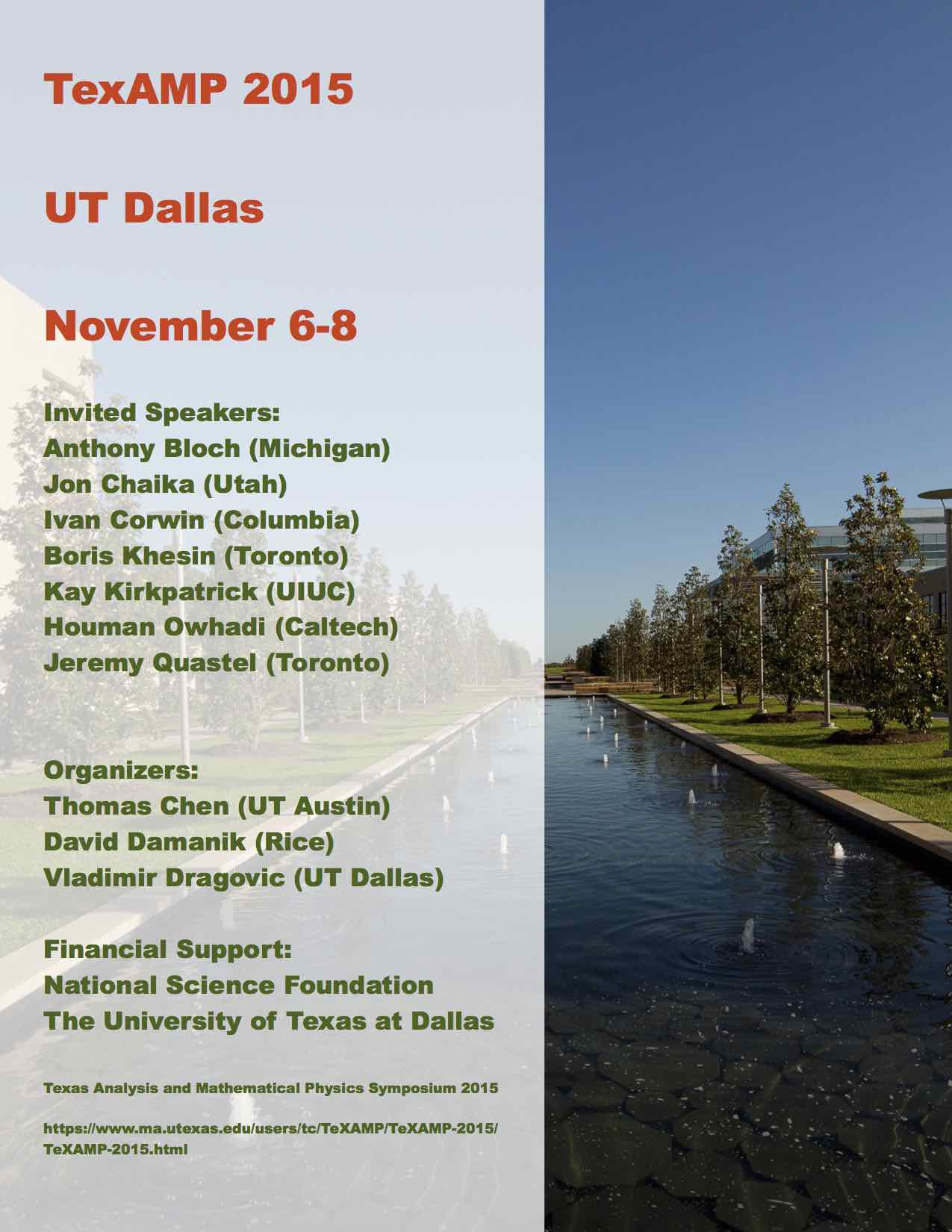# UNIVERSITY OF TEXAS AT DALLAS### Abstracts

 Anthony Bloch (University of Michigan): Toda flows, gradient flows and the generalized Flaschka map In this talk I will describe the geometry of the integrable Toda lattice flows and some their generalizations. In particular I will discuss their gradient-like nature and relationship with other gradient flows on manifolds. I will also discuss a generalization of the Flaschka map which is used analyze integrability and describe its geometry in a Lie theoretic setting. Jon Chaika (University of Utah): On "circle" averages on translation surfaces Consider a flat torus. If you flow at a fixed rate simultaneously in every direction from a fixed point, you will get a sequence of "circles" that equidistribute on that surface. Translation surfaces are generalizations of tori and one can ask the same question. Flow for time T in all directions from p. Do these "circles" equidistribute? This talk will present progress on this problem: For almost every surface (with respect to any SL(2,R) invariant measure) the answer is yes except possibly on a set of density 0 (that can depend on the point). The full question remains open and I'll state some other open problems. Relevant terminology will be defined. This is joint work with P. Hubert. Ivan Corwin (Clay Mathematics Institute, Columbia University): Integrable probability and the KPZ universality class The Gaussian central limit theorem says that for a wide class of stochastic systems, the bell curve (Gaussian distribution) describes the statistics for random fluctuations of important observables. In this talk I will look beyond this class of systems to a collection of probabilistic models which include random growth models, particle systems, optimization problems, matrices and stochastic PDEs. I will explain how through certain "integrable" or "exactly solvable" examples we are able to see how these different examples all fall into a single universality class -- the KPZ class -- with a much richer mathematical structure than that of the Gaussian. This talk is expository and meant for a wide audience without any background in these areas. Boris Khesin (University of Toronto): Pentagram maps and nondegenerate curves A plane curve is called nondegenerate if it has no inflection points. How many classes of closed nondegenerate curves exist on a sphere? We are going to see how this geometric problem, solved in 1970, reappeared along with its generalizations in the context of the Korteweg-de Vries and Boussinesq equations. Its discrete version is related to the 2D pentagram map defined by R.Schwartz in 1992. We will also describe its generalizations, pentagram maps on polygons in any dimension and discuss their integrability properties. This is a joint work with Fedor Soloviev. Kay Kirkpatrick (UIUC): Bose-Einstein condensation: From many quantum particles to a quantum "superparticle" Near absolute zero, a gas of quantum particles can condense into an unusual state of matter, called Bose-Einstein condensation (BEC), that behaves like a giant quantum particle. The rigorous connection has recently been made between the physics of the microscopic many-body dynamics and the mathematics of the macroscopic model, the cubic nonlinear Schrodinger equation (NLS). I'll discuss progress with Gerard Ben Arous and Benjamin Schlein on a central limit theorem for the quantum many-body dynamics, a step towards large deviations for Bose-Einstein condensation. Houman Owhadi (Caltech): Computational Information Games We show how the discovery/design of scalable numerical solvers can be addressed/automated as a Uncertainty Quantification/Decision Theory problem by reformulating the process of computing with partial information and limited resources as that of playing underlying hierarchies of adversarial information games. As an illustration we show how the application of the proposed approach to the resolution of elliptic PDEs with rough ($L^\infty$) coefficients leads to a near-linear complexity multigrid/multiresolution method with rigorous a-priori accuracy and performance estimates. In this application, the numerical solver is discovered by identifying optimal strategies for gambling on the value of the solution of the PDE based on hierarchies of nested measurements of its solution or source term. Jeremy Quastel (University of Toronto): The Kardar-Parisi-Zhang equation and universality class We will survey some of the recent advances on the KPZ equation, in particular on the weak and strong universality conjectures. Return to main page